## Breadth First Search Algorithm | BFS Example

• Breadth First Search or BFS is a graph traversal algorithm.
• It is used for traversing or searching a graph in a systematic fashion.
• BFS uses a strategy that searches in the graph in breadth first manner whenever possible.
• Queue data structure is used in the implementation of breadth first search.

## BFS Example-

Consider the following graph-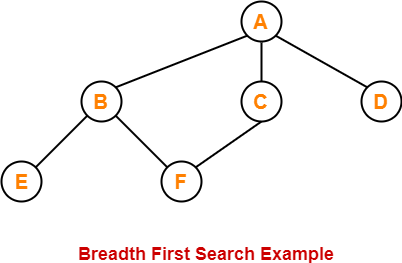The breadth first search traversal order of the above graph is-

A, B, C, D, E, F

#### BFS (V,E,s)

for each vertex v in V – {s}

do

color[v] ← WHITE

d[v] ← ∞

π[v] ← NIL

color[s] = GREY

d[s] ← 0

π[s] ← NIL

Q ← { }

ENQUEUE (Q,s)

While Q is non-empty

do v ← DEQUEUE (Q)

for each u adjacent to v

do if color[u] ← WHITE

then color[u] ← GREY

d[u] ← d[v] + 1

π[u] ← v

ENQUEUE (Q,u)

color[v] ← BLACK

## Explanation-

The above breadth first search algorithm is explained in the following steps-

## Step-01

Create and maintain 3 variables for each vertex of the graph.

For any vertex ‘v’ of the graph, these 3 variables are-

### 1. color[v]-

• This variable represents the color of the vertex v at the given point of time.
• The possible values of this variable are- WHITE, GREY and BLACK.
• WHITE color of the vertex signifies that it has not been discovered yet.
• GREY color of the vertex signifies that it has been discovered and it is being processed.
• BLACK color of the vertex signifies that it has been completely processed.

### 2. Π[v]-

This variable represents the predecessor of vertex ‘v’.

### 3. d[v]-

This variable represents the distance of vertex v from the source vertex.

## Step-02

For each vertex v of the graph except source vertex, initialize the variables as-

• color[v] = WHITE
• π[v] = NIL
• d[v] = ∞

For source vertex S, initialize the variables as-

• color[S] = GREY
• π[S] = NIL
• d[S] = 0

### Step-03

Now, enqueue source vertex S in queue Q and repeat the following procedure until the queue Q becomes empty-

1. Dequeue vertex v from queue Q.

2. For all the adjacent white vertices u of vertex v,

do-

color[u] = GREY

d[u] = d[v] + 1

π[u] = v

Enqueue (Q,u)

3. Color vertex v to black.

## BFS Time Complexity-

The total running time for Breadth First Search is O (V+E).

## Problem-

Traverse the following graph using Breadth First Search Technique-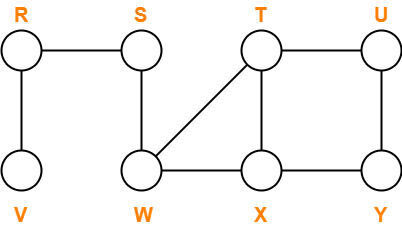Consider vertex S as the starting vertex.

## Solution-

### Step-01:

For all the vertices v except source vertex S of the graph, we initialize the variables as-

• color[v] = WHITE
• π[v] = NIL
• d[v] = ∞

For source vertex S, we initialize the variables as-

• color[S] = GREY
• π[S] = NIL
• d[S] = 0

We enqueue the source vertex S in the queue Q.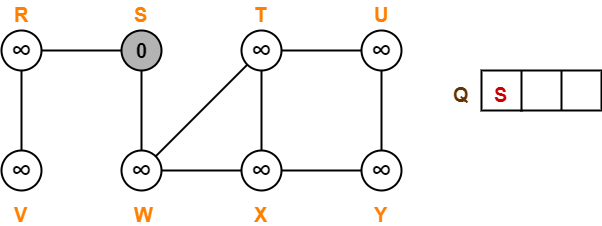### Step-02:

• Dequeue vertex S from the queue Q
• For all adjacent white vertices ‘v’ (vertices R and W) of vertex S, we do-

1. color[v] = GREY
2. d[v] = d[S] + 1 = 0 + 1 = 1
3. π[v] = S
4. Enqueue all adjacent white vertices of S in queue Q

• color[S] = BLACK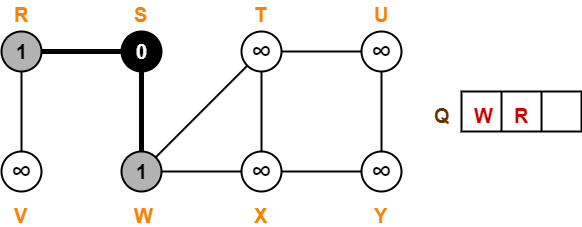### Step-03:

• Dequeue vertex W from the queue Q
• For all adjacent white vertices ‘v’ (vertices T and X) of vertex W, we do-

1. color[v] = GREY
2. d[v] = d[W] + 1 = 1 + 1 = 2
3. π[v] = W
4. Enqueue all adjacent white vertices of W in queue Q

• color[W] = BLACK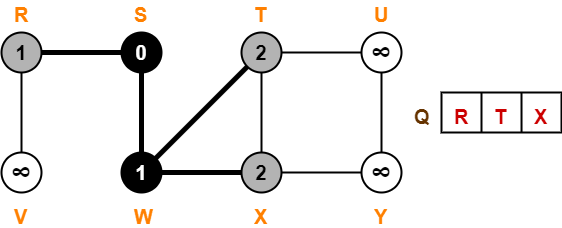### Step-04:

• Dequeue vertex R from the queue Q
• For all adjacent white vertices ‘v’ (vertex V) of vertex R, we do-

1. color[v] = GREY
2. d[v] = d[R] + 1 = 1 + 1 = 2
3. π[v] = R
4. Enqueue all adjacent white vertices of R in queue Q

• color[R] = BLACK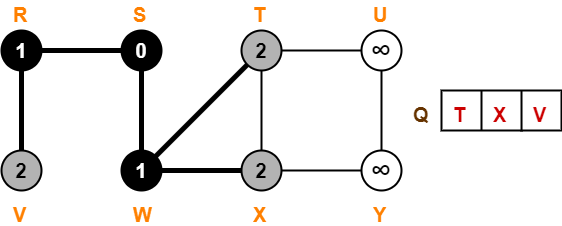### Step-05:

• Dequeue vertex T from the queue Q
• For all adjacent white vertices ‘v’ (vertex U) of vertex T, we do-

1. color[v] = GREY
2. d[v] = d[T] + 1 = 2 + 1 = 3
3. π[v] = T
4. Enqueue all adjacent white vertices of T in queue Q

• color[T] = BLACK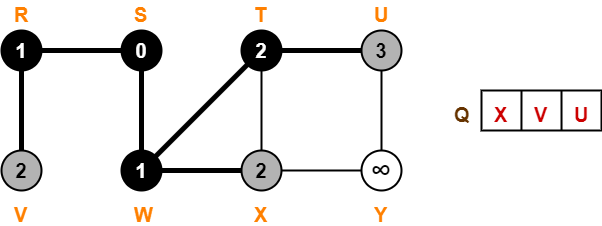### Step-06:

• Dequeue vertex X from the queue Q
• For all adjacent white vertices ‘v’ (vertex Y) of vertex X, we do-

1. color[v] = GREY
2. d[v] = d[X] + 1 = 2 + 1 = 3
3. π[v] = X
4. Enqueue all adjacent white vertices of X in queue Q

• color[X] = BLACK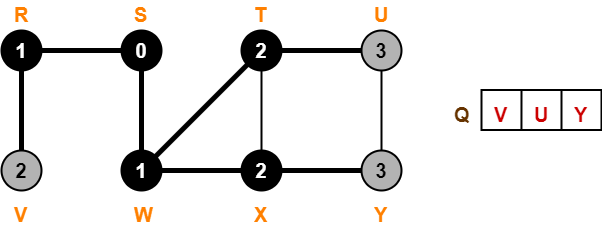### Step-07:

• Dequeue vertex V from the queue Q
• There are no adjacent white vertices to vertex V.
• color[V] = BLACK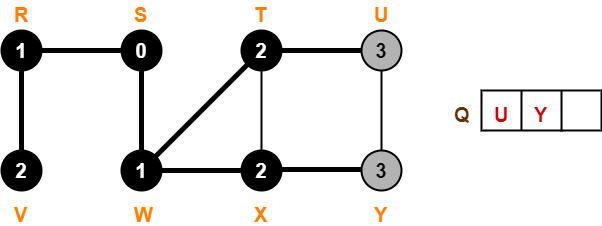### Step-08:

• Dequeue vertex U from the queue Q
• There are no adjacent white vertices to vertex U.
• color[U] = BLACK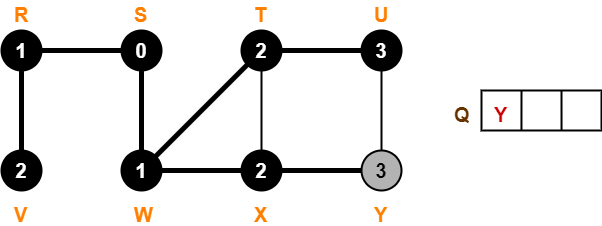### Step-09:

• Dequeue vertex Y from the queue Q
• There are no adjacent white vertices to vertex Y.
• color[Y] = BLACK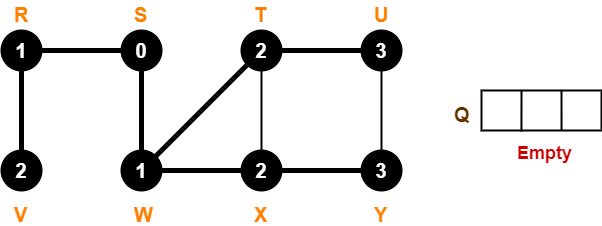Since, all the vertices have turned black and the queue has got empty, so we stop.

This is how any given graph is traversed using Breadth First Search (BFS) technique.